# Improving the Energy Efficiency in Mobile Ad-Hoc Network Using Learning-Based Routing

Improving the Energy Efficiency in Mobile Ad-Hoc Network Using Learning-Based Routing

Vijay Vasanth Aroulanandam Thamarai Pugazhendhi Latchoumi Karnan BalamuruganTiruchengode Lakshmanasamy Yookesh

Department of Computer Science and Engineering, Vel Tech Rangarajan Dr. Sagunthala R&D Institute of Science and Technology, Chennai 600062, India

Department of Computer Science and Engineering, VFSTR (Deemed to be University), AP 522213, India

Department of Mechanical Engineering, VFSTR (Deemed to be University), AP 522213, India

Division of Mathematics, Department of Science and Humanities, VFSTR (Deemed to be University), AP 522213, India

Corresponding Author Email:
Page:
337-343
|
DOI:
https://doi.org/10.18280/ria.340312
15 February 2020
|
Accepted:
2 May 2020
|
Published:
30 June 2020
| Citation

OPEN ACCESS

Abstract:

Improving Mobile Ad-Hoc Network (MANET) performance is a tedious task because of the dynamic and uncertain characteristics of the nodes. MANET nodes connected to multiple applications that involve a high exchange of data. To add reliability to the application services, MANETs optimized through dedicated load balancing schemes. The Dynamic Range Clustering (DRC) algorithm associated with Learning-based Routing (LR) used in the present work to improve the energy efficiency and the nature of the network's data handling. DRC design focuses on selecting the head of the cluster and maintaining stability over the cluster. LR selects unique neighbors that assist in ensuring the efficient, non-congested communication of data exchange. An effort has made to combine the two incompatible methods to increase the performance of the network over differential network traffic. The proposed approach tested using simulations and measures output by comparative analysis.

Keywords:

learning-based routing, neural networks, node range adjustment, sequential learning, weighted clustering

1. Introduction

Within a MANET, the most common wireless media interlocks the self-disciplined mobile host groups for communication. The host nodes separated by the physical distance to which the multi-hop connections used. This network designed for unique applications that don't depend on any backbone infrastructure . With MANETs rapid development technology, it employed industrial, residential, military, and science applications in real-time. The positive position of this network was operating with fewer resources in an emergency scenario would also serve as a temporary backup. The nodes/mobile hosts are dynamic and move around freely on encompassing the area that permits the network to cover the entire region. As mentioned earlier, the nodes rely on their neighbors for multi-hop communications over the spread region.

With the mobility feature, the nodes can maintain and restructure of the network topology . Communication involves data transmission between one-hop and multi-hop neighbors that was accomplished using routing and transmission protocol. The nodes, which encompass a wide region, are dependent on their neighbors for data transmission and receiving . The confronted MANETs weakness was the absence of a central controller and its autonomous character. They have a significant effect on the daily process of node contact and networking. Scaling the nodes in the network was also tedious because of built-in mobility which causes repeated connection breakage and data loss .

The systematic clustering methodology used to address the various issues related to data transmission and scalability. As per the call of the researcher, clustering helps in network stability through correlating links and communication through a hierarchy. The encompassing nodes are segregated as small enclosures called clusters, each attempt to hold two different node types . The Cluster Head (CH) and the Cluster Member (CM) are the two categories of nodes in the interconnected groups/enclosures. CHs are responsible for communication, scheduling, medium allocation, and aggregation for the maintenance of network endurance . The size of the cluster was always different and depends on the number of neighbors who make requests. The range of communication of the cluster depends on the radio range of the CH. The battery power of the nodes was maintained in a less density cluster, while the cluster energy was exhausted at an early stage in the case of a high-density cluster . The dynamic nature of the network, the excessive exchange of information, and the stability of the network has been controlled by the cluster head with better resource utilization. In other words, clustering improves network scalability, resource utilization, and seamless connectivity despite network coverage. Clusters distribute tasks across the network to prevent overloading at the node level and to harmonize communications. Routing endurance and path set up in a clustering environment minimizes the impact of bottlenecks to achieve optimal network performance .

To achieve stability, unpredictable network traffic communicates between single and multi-track nodes increased network traffic requires a formal load balancing scheme to effectively distribute incoming data between available routes . Load balancing was intended to enhance the use of resources by the network controlling exploitation. Multi-path communication improves communication rates with increased bandwidth accessibility . Effective load balancing focuses on estimating and disseminating incoming traffic.

2. Related Works

In wireless sensor networks, a node placement method was proposed for load balancing. By restricting incoming network traffic, this approach allows minimal-hop data transmission. In comparison, this node placement strategy was cost-effective and energy-efficient, and it fails to represent the number of control messages exchanged .

Delay aware load balancing technique was developed to reduce the wireless network adjournment and packet segregation errors. Through analyzing the traffic and packet flow to evaluate the sequence of the packet to be transmitted, this algorithm best suited for multi-path communication .

The authors proposed a clustering scheme with a self-organizing attribute to ensure scalability and make the network stable. This clustering was inspired by birds flocking behavior to dynamically adjust cluster size to minimize congestion. This clustering works best in Group mobility-induced MANETs . They ended up with a result that guaranteed node durability and robustness with lower energy requirements.

The load balancing would predict the optimal route by using Ant Colony Optimization (ACO) to find appropriate pathways. The path preferences were determined using metrics such as delay, bandwidth, and accessibility to the neighbor. ACO technique has become an effective method to enhance network performance, and it does not limit the exchange of control messages when the number of paths increases .

A generic clustering algorithm  was indeed designed to improve the stability of group-based networks and the cluster lifetime. The algorithm developed was based on the density of the nodes and the alliance between them. The writers in [19, 20] suggest a central controller that had already implemented semi-matching load balancing for WLAN. To spread the uneven network loads, the central controller acquires knowledge of the active channel time. The matching process was performed using the Kuhn-Munkres (K-M) algorithm to maximize network throughput and this method was least reliable for multi-rate WLANs problems .

3. Proposed Method

3.1 Network model

In a distributed X*Y dimension network, the mobile nodes are defined by {n (1,) n (2), etc. The nodes share the information among themselves using wireless links (V). If the network was represented as a graph G then, G = (N, V) would define node sets and links.

A node ‘i’ has a wireless link with its neighbor ‘j’ if dist (i, j) < R (i)(j), then R is the ratio within the I or j range.

The nodes in the network are grouped into clusters whereby the CH, pursued by its CM, guided every cluster. The CH aggregates its CM data and ensures distribution at the Base Station (BS). Figure 1 shows a sample cluster network.

## 2.1.png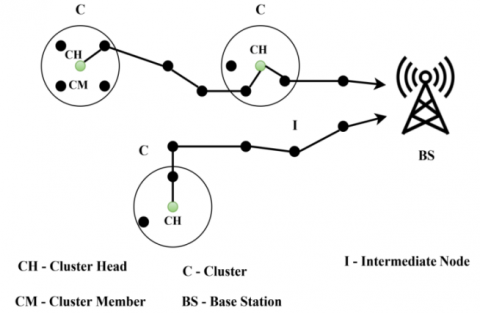Figure 1. The proposed cluster network model

3.1.1 Assumptions

1. Intermediate nodes are not part of any cluster but a member of X*Y.

2. If the CH is present in one-hop, it communicates directly with the BS; otherwise, it relies on its neighbors for contact.

3. The initial CH is the first communicating node, and the later CH is chosen based on appropriate constraints.

## 2.2.png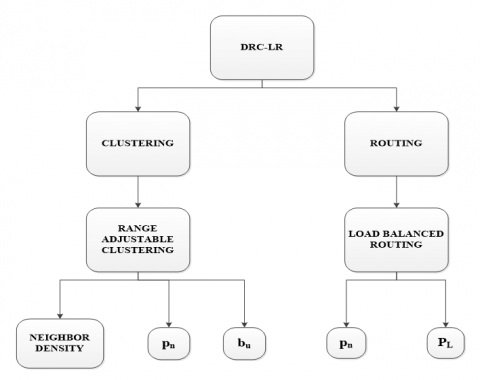Figure 2. DRC-LR process

3.2 Dynamic range clustering (DRC) with learning-based routing (LR) scheme

The proposed DRC-LR is to increase the ability of MANET nodes to handle the load. Figure 2 illustrates the overall DRC-LR process.

3.3 Dynamic range clustering (DRC) algorithm

DRC makes a collection of CH simpler across a set of learning processes. A neural network evaluation for the option of specific CH affects the learning process. Besides, the learning process determines the number of optimum CM required to relay the current network traffic.

Two explicit and hidden constraints are executed for each of the learning iterations performed by neural network evaluation. The two explicit constraints are assessed using neighboring density and use of bandwidth. For several packets, the implicit constraint decides the range of the current CH. Previously, the head of the cluster is chosen using the variable weight function (∇w) derived from the neural computation output.

The weight function to pick a CH needs only specific metrics of the constraints that is because the CH node acts as the aggregator that defines a specific maximization. As the number of active transmitting nodes is unknown, the parametric cannot be predicted at the initial stage.

Let vc, tt and tr be the connection capacity, transmit time and receipt time of a node n, then use Eq. (1) to calculate the bandwidth utilization of the node wire bu for m transmissions.

$\mathrm{b}_{\mathrm{u}}=\mathrm{v}_{\mathrm{c}} *\left(1-\frac{\mathrm{t}_{\mathrm{r}}-\mathrm{t}_{\mathrm{t}}}{\mathrm{t}_{\mathrm{t}}}\right), \forall \mathrm{m}$       (1)

The number of node-associated neighbors determines its density $(\rho)$, which is estimated using Eq. (2).

$\rho=\left|\left\{V_{i j} \in N_{n}: \operatorname{dist}(i, j) \leq R\right\}\right|$      (2)

The bandwidth utilization of the node must be lower for incoming network traffic, while the density must be high for rapid switching. The above factors result in inequality in the decision of the CH node; therefore, the variable weight function $(\nabla w)$ is imposed on the above functions to resolve the inequality. Eqns. (3) and (4) are used to calculate the weight by acquiring the above factors.

$\nabla w=\sum_{j=1}^{n} V_{i j} * \sigma\left(n_{j}\right)+b_{u}$      (3)

where,

$\sigma(\mathrm{n})=\frac{\mathrm{e}^{\rho(\mathrm{n})}-\mathrm{e}^{-\rho(\mathrm{n})}}{\mathrm{e}^{(\mathrm{n})}+\mathrm{e}^{-\rho(\mathrm{n})}}$ and $\mathrm{V}_{\mathrm{ij}} \in\{0,1\}$      (4)

The weight function is estimated to occur as a transigmoid function due to a set of observations. The higher node is considered to be the cluster header. The selected CH is the absolute neuron of the current observation set; the neuron is subject to change based on its effectiveness as decided to use Corollary 1. After the CH is selected, it sends a request for transmission to all its CMs. This request may be received by the active nodes that initiate transmission. The CH calculates the number of packets and checks whether the available bandwidth is sufficient to transmit them. If the available bandwidth is sufficient, the CH determines the number of CMs (including transmitting nodes) required for communication. In this case, the communication range of the CH is variable. The range is variable between the maximum coverage of the node and the initial CH range. The range cannot be reduced below the initial configuration measures for the node. The neural computing process for the selection of CH is shown in Figure 3.

## 2.3.png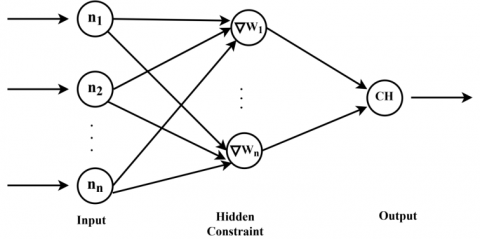Figure 3. Neural computation process for header selection

3.3.1 Corollary 1: Considering the energy expenditure of the CH as its dependence factor

Analysis 1: Let us consider the problem of energy optimization as a linear bounded solution. The initial node energy (Einit) will not remain the same if it has utilized its energy for transmitting (Etx)and receiving (Erx) packets. The remaining node energy (Ene) is the dependency factor of a node. Ene = Einit – (Etx +Erx) needs to be estimated for the CH over each aggregation process.

The energy of the node cannot be regenerated for which the variable range is used as a solution. The number of nodes to be used at time t is to estimate the average energy of the cluster with the current node density. The average energy of the cluster members is calculated using Eq. (5).

$E_{n e}(a v g)=\sum_{i=1}^{n} \frac{E_{n e}(i)}{n}, i \neq C H$     (5)

If the average energy is higher than CH’s Ene, then the learning process is re-installed. In a re-installed learning process, the last CH is discarded as it does not have enough energy. Additional verification of the energy level is not necessary for this learning process. The new CH selected will re-center its range of communication, where n = n-1 will be the number of CMs before R variance.

The range and variance of the CH depend on the number of nodes involved in the communication. If the whole CM or the longest in-cluster CM is involved in the transmission process, the range does not need to be changed and therefore the connectivity at the time (t+x) remains the same.

## 2.4.png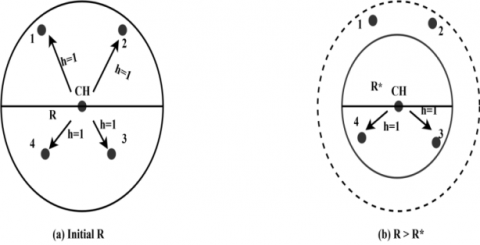Figure 4. Range variance illustrations

The initial value of the Connectivity cn is calculated using Eq. (6).

$c_{n}=\frac{\sum v_{i j}}{n(n-1)}$      (6)

If $\operatorname{dist}(\mathrm{i}, \mathrm{j})<R(\mathrm{i}) \| \mathrm{R}(\mathrm{j})$ is true then vij=1 else 0. If only a minimum number of CM participates in the communication, then R of the CH is minimized to serve the incurring communicating nodes.

In that case, the new ρ of the CH is updated and the transmitted energy is allocated for the current node density. The minimum and maximum hops up to the point where the CH will use energy are 1. The nature of the estimation of the hop count in a variable R is shown in Figure 4.

3.4 Learning-based routing (LR) scheme for efficient load dissemination

As discussed in the earlier section, inadequate or defective load handling results in the network resources and output being degraded. Learning-based routing is proposed to reduce the disadvantages of data dissemination. No additional computation is required in the learning-based routing scheme; packet count, Pn from the CH is considered to further communicate with the available neighbors. The probability of packet loss $\emptyset\left(p_{L}\right)$ of the forwarding, the node needs to be calculated for the transmitted position pn. The number of packets and the likelihood of drop are calculated, respectively, using Eqns. (7) and (8).

$p_{n}=\frac{d_{r}}{v_{t}}$      (7)

where, dr and vt are the data rate and link time.

$\emptyset\left(p_{L}\right)=p_{n \max } *\left(\frac{q_{a}-q_{m i n}}{q_{m a x}-q_{m i n}}\right)$      (8)

where, pnmax is the maximum number of packets transmitted and qa, qmin, and qmax are the average, minimum, and maximum queue usage.

The learning-based routing model selects a node with a lesser drop probability that sustains maximum pn.

3.4.1 Learning 1: If pn increases, qa is greater than qmax, resulting in packet loss

Solution 1: When qa > qmax, then Equation (8) can be written as $\emptyset\left(p_{L}\right)=p_{\text {nmax }} *\left(\frac{q_{a}-q_{\min }}{q_{a}-q_{m i n}}\right)=p_{\text {nmax }}$. In this case, if qmax<pn, then overflow occurs and for all unattended transmissions, the incoming traffic is disintegrated in the same node. Therefore, the loss probability is always 1. The learning phase is keen about minimizing loss by determining nodes that are capable of handling pn<qa. If the maximum queue size is considered, then the free space in the queue is unknown resulting in non-profit neighbor selection.

3.4.2 Learning 2: qmin≤qa

Solution 2: If the above condition is achieved, then the intermediate forwarder will have better queue size to relay information to the base station. Therefore, in this analysis, Eq. (8) can be expressed as $\emptyset\left(\mathrm{p}_{\mathrm{L}}\right)=\mathrm{p}_{\mathrm{nmax}} *\left(\frac{\mathrm{q}_{\mathrm{a}}}{\mathrm{q}_{\mathrm{max}}}\right), \mathrm{q}_{\mathrm{a}} \neq \mathrm{q}_{\mathrm{max}}$.

The number of forwarders with this condition cannot be concurrently used, as the multipath transmission is restricted. Therefore, for ‘m’ transmissions, a scheduling process needs to be renowned to prevent congestion free transmission. Hence, a transmission matrix is defined with the above constraints for this learning process. The transmission factor is defined with the data rate observed from the serving CH.

The number of packets that are inconsistent with the transmission will be estimated using discriminate metric ∆δ. The data rate is mapped to the packet loss probability as an inverse function for which ∆δ must be computed for a set of m transmissions. The relationship between the transmission factor and qa is represented as in Eq. (9).

$\left(\begin{array}{c}\frac{1}{\mathrm{d}_{\mathrm{r} 1}} \\ \frac{1}{\mathrm{d}_{\mathrm{r} 2}} \\ \vdots \\ \frac{1}{\mathrm{d}_{\mathrm{rm}}}\end{array}\right)\left(\begin{array}{c}\emptyset \mathrm{p}_{\mathrm{L} 1} \\ \emptyset \mathrm{p}_{\mathrm{L} 2} \\ \vdots \\ \emptyset \mathrm{p}_{\mathrm{Lm}}\end{array}\right)=\frac{1}{\Delta \delta}\left(\begin{array}{c}\mathrm{q}_{\mathrm{a} 1} \\ \mathrm{q}_{\mathrm{a} 2} \\ \vdots \\ \mathrm{q}_{\mathrm{am}}\end{array}\right)$      (9)

Eq. (7) and Eq. (8) are used to expand Eq. (9) and are represented as Eq. (10).

$\left(\begin{array}{c}\frac{1}{\mathrm{p}_{\mathrm{n} 1} * \mathrm{v}_{\mathrm{t} 1}} \\ \frac{1}{\mathrm{p}_{\mathrm{n} 2} * \mathrm{v}_{\mathrm{t} 2}} \\ \vdots \\ \frac{1}{\mathrm{p}_{\mathrm{nm}} * \mathrm{v}_{\mathrm{tm}}}\end{array}\right)\left(\begin{array}{c}\mathrm{p}_{\mathrm{n} 1} *\left(\frac{\mathrm{q}_{\mathrm{a} 1}}{\mathrm{q}_{\mathrm{max} 1}}\right) \\ \mathrm{p}_{\mathrm{n} 2} *\left(\frac{\mathrm{q}_{\mathrm{a} 2}}{\mathrm{q}_{\mathrm{max} 2}}\right) \\ \vdots \\ \mathrm{p}_{\mathrm{nn}} *\left(\frac{\mathrm{q}_{\mathrm{an}}}{\mathrm{q}_{\mathrm{maxm}}}\right)\end{array}\right)=\frac{1}{\Delta \delta}\left(\begin{array}{c}\mathrm{q}_{\mathrm{a} 1} \\ \mathrm{q}_{\mathrm{a} 2} \\ \vdots \\ \mathrm{q}_{\mathrm{a}m}\end{array}\right)$       (10)

The generalized normal form of Eq. (11) is simplified as follows:

$\left(\frac{1}{p_{n} * v_{t}}\right)\left(p_{n} *\left(\frac{q_{a}}{q_{m a x}}\right)\right)=\frac{1}{\Delta \delta}\left(q_{a}\right)$      (11)

The discriminate metric is computed as Eq. (12) by solving Eq. (11).

$\Delta \delta=v_{t} * q_{\max }$       (12)

After the completion of the transmission process, the node is categorized as learning 1 or 2. The node classified under Learning 2 is optimal and further transmission is pursued. The learning process is repeated until the next node leads to the BS. Similarly, the learning process is updated before the next communication begins.

If the node fails to learn 2, the next set of neighbors will be identified through a news broadcast. In the meantime, if the nodes $\Delta \delta$ are satisfied with the incoming network traffic, the bu adjustment concerning the neighbor density shall be made to accept the current communication set. A neighbor who does not satisfy a discriminatory metric is excluded from participating in the transmission process.

4. Performance Analysis

The performance of the proposed DRC-LR is evaluated through simulations using a network simulator tool.

The following metrics are estimated for assessment: Delay, Packet Loss, Communication Overhead, Reclustering, and Remaining Energy. Table 1 shows the parameters that are considered along with their values.

Table 1. Simulation parameters and values

 Parameter Value Network Region 1000m x 1000m Number of Mobile Nodes 100 MAC Protocol 802.11 Range 250m Application Type Constant Bit Rate Packet Size 512Kb Einit 16J Pause Time 2 ms Simulation Time 300s Compared with REA-MAODV , QUACS  and EE-MBOC [24, 25]
Notes: REA-MAODV – Real Multicast Ad hoc On-Demand Distance Vector Routing, QUACS – Queuing Ant Colony System, EE-MBOC – Energy Efficient Modified Butterfly Optimization-based Clustering.

4.1 Delay analysis

The comparison of DRC-LR for the delay with existing methods is shown in Figure 5. In the DRC-LR, initial communication at the node level is organized by the CH. Cluster re-formation time is large, so no new procedures are required.

## 5.png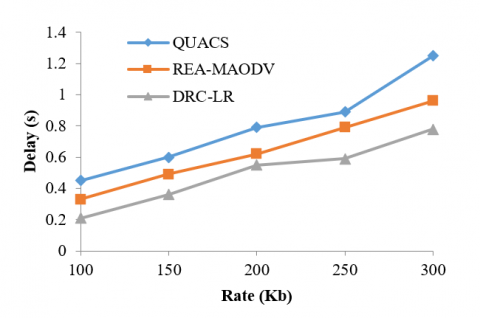Figure 5. Delay comparison

As a result, the time complexity of cluster formation is less. The node with lower packet drops probability is selected in the LR scheme. This minimizes the number of retransmissions due to packet loss. CH aggregation, stable clustering, and efficient neighbor selection are the main reasons for minimizing packet loss. An increased packet rate is handled through selective neighbors to avoid delayed transmission. The proposed DRC-LR results in 37.6% and 23.20% less delay than QUACS and REA-MAODV respectively.

4.2 Packet loss probability

The numbers of transmissions are hidden due to the increase of packet rate and congestion. Unlike other generic methods, ratings and dissemination of data are administered through qa optimized nodes. For each transmission from the CH, qa and qmax of the node are observed.

In the learning phase, the restriction qmin ≤ qa must be met by the node to participate in the transmission. The complete transmission process is based on the queue utilization of the nodes to the bu in the previous transmission. Selecting an effective bu node with a queue constraint improves seamless communication by reducing packet loss and it can be verified from Figure 6.

## 6.png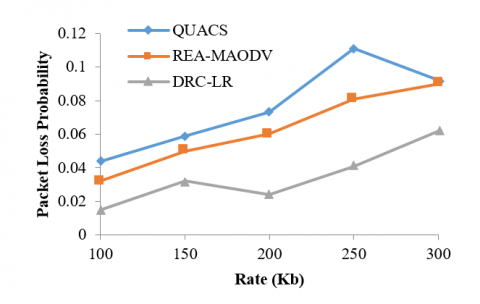Figure 6. Packet loss probability

As a result, the probability of loss of the proposed DRC-LR is 32.60% and 31.11% lower than the existing QUACS and REA-MAODV.

The number of node replacements inside and outside the cluster is restricted using DRC and LR independently. The number of CH-transmissions that can be changed is less within (t+x) due to ∇ω and range variance operation. The intermediate node selection follows a queue-based verification from which a specific neighbor is selected. The need for the replacement of nodes and the discovery of new routes is, therefore lower in the proposed method. This minimizes the frequency of exchange of control messages, reducing overhead communication and it can be verified form Figure 7. DRC-LR requires 46.8% and 37.72% fewer control bits than the existing QUACS and REA-MAODV, which reduces overhead communication.

## 7.png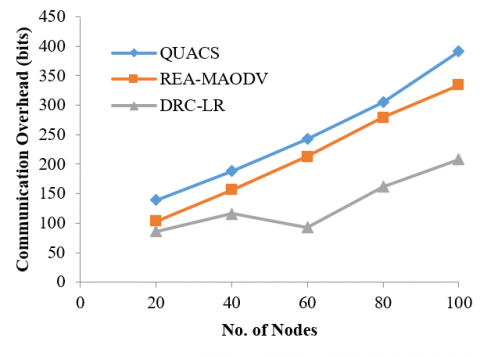4.4 Re-clustering instance

In the proposed DRC-LR, cluster stability is improved by the transigmoid function. This function selects the optimal cluster header by assessing their explicit hidden constraints. Prolonged communication is ensured by using a single CH with a time t > (t+x) range variance. The stability of the cluster is maintained externally by checking pL of the external nodes. From Figure 8, the need for CH replacement at a time (t+x) is, therefore, less than the existing EE-MBOC. Precise neighbor density factor and bandwidth utilization factors determine a reliable CH that sustains long-term communication. Compared to EE-MBOC, the proposed DRC-LR had a 34.17% lower chance of re-clustering.

## 8.png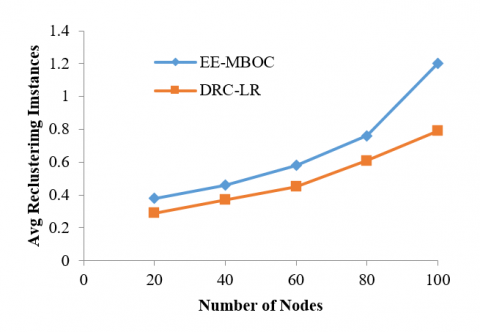Figure 8. Re-clustering instance comparison

4.5 Remaining energy

Energy conservation in the proposed DRC-LR is carried out as a non-linear hidden optimization issue. In this optimization, the node maintains prolonged communication, avoiding autonomous energy expenditure.

## 9.png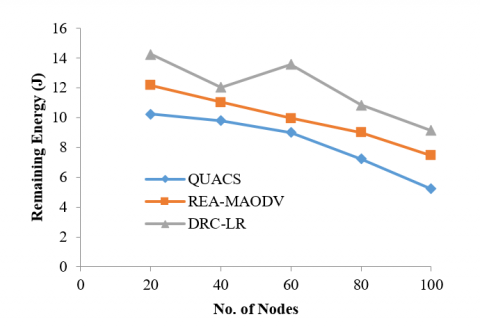Figure 9. Remaining energy comparison

Clustering is an additional benefit in managing the network's energy consumption keeping the (n-1) nodes out; the CH is adjusted if its residual energy is less than the average energy. In a fixed (t+x), the energy expenditure of all the nodes inside the cluster is lower. The average cluster energy retained in this method is high. The energy spent by a single CM is less as the nodes are least or delayed in aggregation. From Figure 9, it is confirmed that the energy stored in the network is strong. The proposed DRC-LR maintains higher remaining energy than QUACS and REA-MAODV where the remaining energy is 42.66% lower and 18.27% lower than the proposed DRC-LR. Table 2 displays the outcome of the simulation executed.

Table 2. Simulation values of DRC-LR compared with QUACS and REA-MAODV

 Parameter QUACS REA-MAODV DRC-LR Delay (s) 1.25 0.96 0.78 Packet Loss Probability 0.092 0.09 0.062 Communication Overhead (bits) 391 334 208 Remaining Energy (J) 5.24 7.47 9.14
5. Conclusion

In MANET load balancing, DRC-LR is proposed to increase reliability. The suggested approach operates in such a way that it enhances the efficiency of the network's data handling. The goal of DRC is to improve the stability of the cluster by selecting an enduring CH to aggregate and disseminate the collected data. Learning-based routing curtails the loss of packets by selecting nodes with a lower probability of loss due to increased network load. The learning process is based on the observations series over every transmission of the network. The integrated method increases network performance by reducing packet loss, delay, overhead contact, and maintaining a significant amount of node residual energy.

Nomenclature
 Kb Kilo bytes ms Microsecond Greek symbols y Vector for all binary variables ω Trainable parameter f Solid volume fraction θ Dimensionless temperature µ Dynamic viscosity, kg. m-1.s-1
References

 Shyam, D., Kot, A., Athalye, C. (2018). Abandoned object detection using a pixel-based finite state machine and single shot multibox detector. IEEE International Conference on Multimedia and Expo (ICME), San Diego, USA, pp. 1-6. https://doi.org/10.1109/ICME.2018.8486464

 Latchoumi, T.P., Jayakumar, L., Latha, P., Janakiraman, S. (2016). OFS method for selecting active features using clustering techniques. In Proceedings of the International Conference on Informatics and Analytics, ACM, Puducherry, India, pp. 1-4. https://doi.org/10.1145/2980258.2982108

 Li, J., Si, Y., Lang, L., Liu, L., Xu, T. (2018). A spatial pyramid pooling-based deep convolutional neural network for the classification of electrocardiogram beats. Applied Sciences, 8(9): 1590. https://doi.org/10.3390/app8091590

 Nazila, M., Raziyeh, H., (2015). A survey on existing mechanisms in energy-aware routing in MANET, International Journal of Computer Applications Technology and Research, 4(9): 673-679. https://doi.org/10.7753/ijcatr0409.1007

 Ezhilarasi, T.P., Dilip, G., Latchoumi, T.P., Balamurugan, K., (2020). UIP-A smart web application to manage network environments, In Proceedings of the Third International Conference on Computational Intelligence and Informatics, pp. 97-108. https://doi.org/10.1007/978-981-15-1480-78

 Sundaranarayan, D., Venkatachalapathy, K. (2018). An effectual load distribution approach based on transmission power and topology controlled clustered environment in mobile Adhoc network. International Journal of Computer Applications, 179(25): 34-38. https://doi.org/10.5120/ijca2018916538

 Khatoon, N., Amritanjali, (2017). Mobility aware energy efficient clustering for MANET: A bio-inspired approach with particle swarm optimization. Wireless Communications and Mobile Computing, 2017: 1-12. https://doi.org/10.1155/2017/1903190

 Linyang, S., Jingbo, S., Jinfeng, D. (2010). A novel energy-efficient approach to DSR based routing protocol for Ad Hoc Network. IEEE International Conference on Electrical and Control Engineering, Wuhan, China, pp. 2618-2620. https://doi.org/10.1109/iCECE.2010.1443

 Mandhare, V.V., Thool, R.C. (2016). Improving QoS of mobile ad-hoc network using cache update scheme in dynamic source routing protocol. Procedia Computer Science, 79: 692-699. https://doi.org/10.1016/j.procs.2016.03.090

 Barati, M.,Atefi, K., Khosravi, F., Daftari, Y.A. (2017). Performance Evaluation of energy efficiency for MANET using AODV routing protocol. IEEE 3rd International Conference on Computational Intelligence and Communication Technology, Kuala Lumpeu, Malaysia, pp. 636-642. https://doi.org/10.1109/ICCISci.2012.6297107

 Latchoumi, T.P., Balamurugan, K., Dinesh, K., Ezhilarasi, T.P. (2019). Particle swarm optimization approach for waterjet cavitation peening. Measurement, 141(6): 184-189. https://doi.org/10.1016/j.measurement.2019.04.040

 Mohammed, A.W., Karibasappa, K. (2012). QoS routing for heterogeneous mobile ad hoc networks, International Journal of Computer Engineering Sciences (IJCES), 2(3): 77-81.

 Li, G., Xu, Y. (2013). A TCP-friendly congestion control scheme for multicast with network coding. Journal of Computational Information Systems, 9(21): 8541-8548. https://doi.org/10.12733/jcis7229

 Chen, L., Heinzelman, W.B. (2005). QoS-aware routing based on bandwidth estimation for mobile ad hoc networks. IEEE Journal on Selected Areas in Communications, 23(3): 561-572.

 Chatterjee, P., Ghosh, S.C., Das, N. (2017). Load balanced coverage with graded node deployment in WSN. IEEE Transactions on Multi-Scale Computing Systems, 3(2): 100-112. https://doi.org/10.1109/TMSCS.2017.2672553

 Delgado, O., Labeau, F., (2017). Delay-aware load balancing over multipath wireless networks. IEEE Transactions on Vehicular Technology, 66(8): 7485-7494. https://doi.org/10.1109/TVT.2017.2655011

 Aftab, F., Zhang, Z., Ahmad, A. (2017). Self-organization based clustering in MANETs using zone-based group mobility. IEEE Access, 5: 27464-27476. https://doi.org/10.1109/ACCESS.2017.2778019

 Selvi, F.A. (2016). Ant based multipath backbone routing for load balancing in MANET. IET Communications, 11(1): 136. https://doi.org/10.1049/iet-com.2016.0574

 Massin, R., Martret, C.J.L., Ciblat, P. (2017). A coalition formation game for distributed node clustering in MANETs. IEEE Transactions on Wireless Communications, 16(6): 3940-3952. https://doi.org/10.1109/TWC.2017.2690419

 Lei, T., Wen, X., Lu, Z., Li, Y. (2017). A semi-matching based load balancing scheme for dense IEEE 802.11 WLANs. IEEE Access, 5: 15332-15339. https://doi.org/10.1109/ACCESS.2017.2733083

 Cheng, Y., Yang, D., Zhou, H. (2018). Det-LB: A load balancing approach in 802.11 wireless networks for industrial soft real-time applications, IEEE Access, 6: 32054-32063. https://doi.org/10.1109/ACCESS.2018.2802541

 Liang, Q.L. (2003). Cluster head election for mobile ad hoc wireless network. 14th IEEE Proceedings on Personal, Indoor and Mobile Radio Communications, 2: 1623-1628. https://doi.org/10.1109/PIMRC.2003.1260389

 Cespedes-Mota, A., Castanon, G., Martínez-Herrer, A.F., Cardenas-Barron, L.E. (2018). Multi objective optimization for a wireless ad hoc sensor distribution on shaped-bounded areas. Mathematical Problems in Engineering, 2018: 1-22. https://doi.org/10.1155/2018/7873984

 Ahmad, M., Ikram, A.A., Wahid, I., Inam, M., Ayub, N., Ali, S. (2018). A bio-inspired clustering scheme in wireless sensor networks: BeeWSN. Procedia Computer Science, pp. 206-213. https://doi.org/10.1016/j.procs.2018.04.031

 Hao, S., Zhang, H., Song, M. (2018). A stable and energy-efficient routing algorithm based on learning automata theory for MANET. Journal of Communications and Information Networks, 3(2): 52-66. https://doi.org/10.1007/s41650-018-0012-7